• 文章目录最小生成树及其性质求解问题总结prim算法应用案例题：数码宝贝拯救者——讨伐恶魔大陆AC代码 最小生成树及其性质 ...①最小生成树是树， 因此其边数等于顶点数减1 , 且树内一定不会有环。 ②对给定的图...
文章目录最小生成树及其性质求解问题总结prim算法：类似Dijkstra（普里姆算法）优缺点应用案例题1：数码宝贝拯救者——讨伐恶魔大陆AC代码题2：问题 A: 还是畅通工程题3：问题 D: 继续畅通工程题4：Freckles思路AC代码题5:问题 E: Jungle Roadskruskal 算法：边贪心策略（克鲁斯卡尔算法"）优缺点应用案例题一：讨伐恶魔大陆
最小生成树及其性质
最小生成树是在一个给定的无向图G(V,E)中求一棵树T, 使得这棵树拥有图G中的所有顶点，且所有边都是来自图G中的边，并且满足整棵树的边权之和最小。
最小生成树有3 个性质需要掌握：
①最小生成树是树， 因此其边数等于顶点数减1 , 且树内一定不会有环。
②对给定的图G(V,E), 其最小生成树可以不唯一， 但其边权之和一定是唯一的。
③由于最小生成树是在无向图上生成的， 因此其根结点可以是这棵树上的任意一个结点。于是，如果题目中涉及最小生成树本身的输出， 为了让最小生成树唯一， 一般都会直接给出根结点， 读者只需以给出的结点作为根结点来求解最小生成树即可。
求解问题总结
给定一个无向图，求其中包含了所有顶点的边权最小之和的树（和为多少，什么样的树）

求解最小生成树一般有两种算法， 即prim算法与kruskal算法。这两个算法都是采用了
贪心法的思想， 只是贪心的策略不太一样。
prim算法：类似Dijkstra（普里姆算法）
优缺点
优点：时间复杂度较低；缺点：思想较难理解
prim算法与Dijkstra算法使用的思想几乎完全相同， 只有在数组d[]的含义上有所区别。
其中，Dijkstra算法的数组d[]含义为起点s到达顶点Vi的最短距离；
而prim算法的数组d[]含义为顶点Vi与集合S的最短距离；
两者的区别仅在于最短距离是顶点Vi针对“起点s”还是 “集合S”。另外，对最小生成树问题而言， 如果仅是求最小边权之和， 那么在prim算法中就可以随意指定一个顶点为初始点， 例如在下面的代码中将默认使用0号顶点为初始点。
根据上面的描述， 可以得到下面的伪代码（注意与prim算法基本思想进行联系）：
//G为图， 一般设成全局变量；数组d为顶点与集合S的最短距离
Prim(G, d[]) {
初始化;
for (循环n次) {
u = 使d[u]最小的还未被访问的顶点的标号;
记u已被访问;
for (从u出发能到达的所有顶点V) {
if (v未被访问&&以u为中介点使得v与集合S的最短距离d[v]更优) {
将G[u][v]赋值给v与集合s的最短距离d[v];
}
}
}
}

和Dijkstra算法的伪代码进行比较后发现，Dijkstra算法和prim算法只有优化d[v]的部分不同，而其他语句都是相同的。这再次说明： Dijkstra算法和prim算法实际上是相同的思路，只不过是数组d[]的含义不同罢了。
应用案例
题1：数码宝贝拯救者——讨伐恶魔大陆
这次亚历山大的任务是讨伐恶魔大陆。和精灵大陆一样， 恶魔大陆也有六个城市， 但是城市的分布与精灵大陆不同， 并且这里城市之间的道路是双向的。图10-44a给出了恶魔大陆的六个城市（V0至V5) 和连接它们的无向边， 边上的数字表示距离（即边权）， 而城市结点的黑色表示还未被攻占。
由于恶魔大陆提前知道了亚历山大要来攻打恶魔大陆， 因此恶魔们事先对所有道路进行了冻结， 希望以此消耗亚历山大的体力去恢复这些道路。不过亚历山大不会坐以待毙， 他在分析恶魔大陆的地图之后打算从防守最薄弱的V0开始进攻，并且使用了“ 爆裂模式” 来对抗他们（图10-44中）。在爆裂模式下， 亚历山大可以随时恢复任意一条已攻占城市所连接的道路， 但是需要消耗那条道路的距离大小的体力（也就是说， 道路有多长， 就需要消耗多少体力去恢复）。并且在恢复某条道路之后， 亚历山大会趁机攻占这条道路所连接的未攻占城市。
为了尽可能节省体力， 亚历山大需要解决这样的一个问题：如何选择需要恢复的道路， 使得
亚历山大可以消耗最少的体力， 并保证他可以攻占所有城市。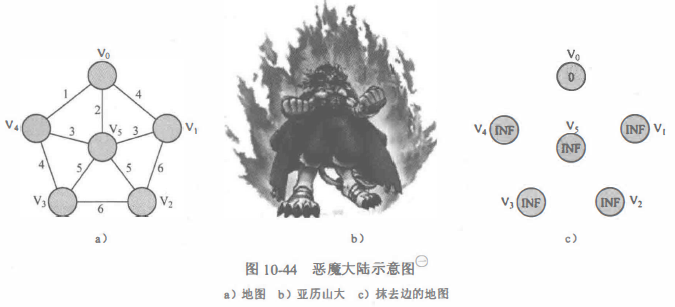这其实就在求一棵最小生成树：
①首先，亚历山大一定是每次从已攻占城市出发去攻打未攻占城市，这说明最后生成的结构一定连通。
②其次， 亚历山大在把V0攻占以后， 总是沿着一条新的道路去攻击一个新的城市， 这说明最后生成的结构的边数一定比顶点数少1。
基于上面两点， 最后生成的结构一定是一棵树（是满足了连通、边数等于顶点数减1)，而亚历山大的要求就是使这棵树的边权之和最小。当然， 如果上面的解释没有看懂的话， 也不妨先记住：这里求的就是一棵以V0为根结点的最小生成树，且接下来亚历山大所做的每一步都是prim算法的步骤。
在这里，亚历山大对地图做出了三个修改：
① 将地图上的所有边都抹去， 只有当攻占一个城市后才把这个城市连接的边显现（这一点和Dijkstra算法中相同）。
②使用“ 爆裂模式” 的能蜇， 将已攻占的城市置于一个巨型防护罩中。亚历山大可以沿着这个防护罩连接的道路去进攻未攻占的城市。
③在地图中的城市Vi (0<=i<=5)上记录城市Vi 与巨型防护罩之间的最短距离（即vi 与每个已攻占城市之间距离的最小值）。由于在①中亚历山大把所有边都抹去了，因此在初始状态下只在城市V0上标记0, 而其他城市都标记无穷大（记为INF, 见图10-44b)。为了方便叙述，在下文中某几处出现的最短距离都是指从城市vi 与当前巨型防护罩之间的最短距离。
输入
第一行输入n：城市数（城市编号为0~n-1）；m：边数；s：起点
接下来m行输入边和边权x,y,z:城市x，y，道路长度z
输出
消耗的最小体力为多少
输入情况：
6 10
0 1 4
0 4 1
0 5 2
1 2 6
1 5 3
2 3 6
2 5 5
3 4 4
3 5 5
4 5 3
输出情况：
15
AC代码
#include<bits/stdc++.h>
using namespace std;
const int INF=1e9;
const int maxv=1000;
map<int,int>adj[maxv];//顶点->边权
int n,m,d[maxv];//n城市数，m道路数，城市号为0~n-1
bool vis[maxv];

void init()
{
for(int i=0;i<n;i++){
vis[i]=false;
d[i]=INF;
adj[i].clear();
}
}
int prim()
{
int ans=0;//存放最小生成树的边权之和
d=0;
for(int i=0;i<n;i++){
int u=-1,MIN=INF;
for(int j=0;j<n;j++){
if(!vis[j]&&d[j]<MIN){
u=j;
MIN=d[j];
}
}
if(u==-1)return -1;
vis[u]=true;
ans+=d[u];//把与集合S距离最短的这个边加入最小生成树
for(map<int,int>::iterator it=adj[u].begin();it!=adj[u].end();it++){
int v=it->first,dis=it->second;
if(!vis[v]&&dis<d[v])
d[v]=dis;
}
}
return ans;
}
int main(){
int x,y,z;
while(scanf("%d",&n)!=EOF){
if(n==0)break;
scanf("%d",&m);
init();
for(int i=0;i<m;i++){
scanf("%d %d %d",&x,&y,&z);
adj[x][y]=adj[y][x]=z;
}
printf("%d\n",prim());
}
return 0;
}


题2：问题 A: 还是畅通工程
http://codeup.cn/problem.php?cid=100000622&pid=0
#include<bits/stdc++.h>
using namespace std;
const int INF = 1e9;
const int maxv = 1000;
map<int, int>adj[maxv];//顶点->边权
int n, m, d[maxv];//n城市数，m道路数，城市号为0~n-1
bool vis[maxv];

void init()
{
for (int i = 1; i<=n; i++){
vis[i] = false;
d[i] = INF;
adj[i].clear();
}
}
int prim()
{
int ans = 0;//存放最小生成树的边权之和
d = 0;
for (int i = 1; i<=n; i++){
int u = -1, MIN = INF;
for (int j = 1; j<=n; j++){
if (!vis[j] && d[j]<MIN){
u = j;
MIN = d[j];
}
}
if (u == -1)return -1;
vis[u] = true;
ans += d[u];//把与集合S距离最短的这个边加入最小生成树
for (map<int, int>::iterator it = adj[u].begin(); it != adj[u].end(); it++){
int v = it->first, dis = it->second;
if (!vis[v] && dis<d[v])
d[v] = dis;
}
}
return ans;
}
int main(){
int x, y, z;
while (scanf("%d", &n) != EOF){
if (n == 0)break;
m=n*(n-1)/2;
init();
for (int i = 0; i<m; i++){
scanf("%d %d %d", &x, &y, &z);
adj[x][y] = adj[y][x] = z;
}
printf("%d\n", prim());
}
return 0;
}


题3：问题 D: 继续畅通工程
http://codeup.cn/problem.php?cid=100000622&pid=3
#include<bits/stdc++.h>
using namespace std;
const int INF = 1e9;
const int maxv = 1000;
struct node{
int w;
int ok;
};
map<int, node>adj[maxv];//顶点->边权
int n, m, d[maxv];//n城市数，m道路数，城市号为0~n-1
bool vis[maxv];

void init()
{
for (int i = 1; i <= n; i++){
vis[i] = false;
d[i] = INF;
adj[i].clear();
}
}
int prim()
{
int ans = 0;//存放最小生成树的边权之和
d = 0;
for (int i = 1; i <= n; i++){
int u = -1, MIN = INF;
for (int j = 1; j <= n; j++){
if (!vis[j] && d[j]<MIN){
u = j;
MIN = d[j];
}
}
if (u == -1)return -1;
vis[u] = true;
ans += d[u];//把与集合S距离最短的这个边加入最小生成树
for (map<int, node>::iterator it = adj[u].begin(); it != adj[u].end(); it++){
int v = it->first;
node dis = it->second;
if (!vis[v]){
if (dis.ok == 1)
d[v] = 0;
else if (dis.w<d[v])
d[v] = dis.w;
}
}
}
return ans;
}
int main(){
int x, y, z, h;
while (scanf("%d", &n) != EOF){
if (n == 0)break;
m = n*(n - 1) / 2;
init();
for (int i = 0; i<m; i++){
scanf("%d %d %d %d", &x, &y, &z, &h);
adj[x][y].w = adj[y][x].w = z;
adj[x][y].ok = adj[y][x].ok = h;
}
printf("%d\n", prim());
}
return 0;
}


题4：Freckles
题目：https://ac.nowcoder.com/acm/problem/115648
In an episode of the Dick Van Dyke show, little Richie connects the freckles on his Dad’s back to form a picture of the Liberty Bell. Alas, one of the freckles turns out to be a scar, so his Ripley’s engagement falls through.
Consider Dick’s back to be a plane with freckles at various (x, y) locations. Your job is to tell Richie how to connect the dots so as to minimize the amount of ink used. Richie connects the dots by drawing straight lines between pairs, possibly lifting the pen between lines. When Richie is done there must be a sequence of connected lines from any freckle to any other freckle.
Input
The input begins with a single positive integer on a line by itself indicating the number of the cases following, each of them as described below. This line is followed by a blank line, and there is also a blank line between two consecutive inputs.
The first line contains 0 < n ≤ 100, the number of freckles on Dick’s back. For each freckle, a line follows; each following line contains two real numbers indicating the (x, y) coordinates of the freckle.
Output
For each test case, the output must follow the description below. The outputs of two consecutive cases will be separated by a blank line.
Your program prints a single real number to two decimal places: the minimum total length of ink lines that can connect all the freckles.
Sample Input
1
3
1.0 1.0
2.0 2.0
2.0 4.0
Sample Output
3.41
思路
题目的意思就是有n个顶点（雀斑），要求用最少的墨水将所有雀斑都连在一起
理解为：把所有雀斑都连接在一起成为无向图，边权就是边的长度，求最小生成树
步骤：

输入所有点的x,y坐标
遍历所有两个点的组合所构成的线（n个顶点共能组成n*(n-1)/2个边）

 for(int i=0; i<n; i++) {
for(int j=i+1; j<n; j++)
adj[i][j]=adj[j][i]=compute(i,j);
}


计算边长（知道两个点的坐标计算边长）

double compute(int a,int b) { //沟谷，知两直角边求斜边
return sqrt(pow((x[a]-x[b]),2)+pow((y[a]-y[b]),2));
}


prime算法
**注意：**输出格式是要每组答案之间留一空行，但最后没有空行
For each test case, the output must follow the description below. The outputs of two consecutive cases will be separated by a blank line.

AC代码
#include<cstdio>
#include<iostream>
#include<cmath>
#include<cstring>
#include<map>
using namespace std;
const double INF = 1e17;
const double eps=1e-9;
const int maxv = 1000;
double x[maxv],y[maxv];//(0~n-1)雀斑的x,y坐标
map<int,double>adj[maxv];//点（id1,id2）->长度
int n;
double d[maxv];
bool vis[maxv];

void init() {
for(int i=0; i<n; i++) {
vis[i]=false;
d[i]=INF;
}
}
double compute(int a,int b) { //沟谷，知两直角边求斜边
return sqrt(pow((x[a]-x[b]),2)+pow((y[a]-y[b]),2));
}
void scan() {
init();
for (int i = 0; i<n; i++)
scanf("%lf %lf",&x[i],&y[i]);
for(int i=0; i<n; i++) {
for(int j=i+1; j<n; j++)
adj[i][j]=adj[j][i]=compute(i,j);
}
}
double prime() {
double ans=0;
d=0;
for(int i=0; i<n; i++) {
int u=-1;
double MIN=INF;
for(int j=0; j<n; j++) {
if(!vis[j]&&(d[j]-MIN)<eps) {
MIN=d[j];
u=j;
}
}
if(u==-1)
return -1;
vis[u]=true;
ans+=d[u];
for(map<int,double>::iterator it=adj[u].begin(); it!=adj[u].end(); it++) {
int v=it->first;
double dis=it->second;
if(!vis[v]&&(dis-d[v])<eps)
d[v]=dis;
}
}
return ans;
}
int main() {
double x, y;
int sum;
scanf("%d",&sum);
while (sum--) {
scanf("%d", &n);
scan();
printf("%.2lf\n",prime());
if(sum)
printf("\n");
}
return 0;
}


题5:问题 E: Jungle Roads
http://codeup.cn/problem.php?cid=100000622&pid=4
#include<bits/stdc++.h>
using namespace std;
const int INF = 1e9;
const int maxv = 50;
map<int, int>adj[maxv];
int n, d[maxv];
bool vis[maxv];

void init() {
for (int i = 1; i <= n; i++){
d[i] = INF;
vis[i] = false;
adj[i].clear();
}
}
void scan() {
init();
int x, y, z, m;
char t;
for (int i = 1; i<n; i++){
getchar();
scanf("%c %d", &t, &m);
x = (int)(t - 'A' + 1);
while (m--){
scanf(" %c %d", &t, &z);
y = (int)(t - 'A' + 1);
adj[x][y] = adj[y][x] = z;
}
}
}
int prime() {
int ans = 0;
d = 0;
for (int i = 1; i <= n; i++) {
int u = -1, MIN = INF;
for (int j = 1; j <= n; j++) {
if (!vis[j] && d[j]<MIN) {
MIN = d[j];
u = j;
}
}
if (u == -1)
return -1;
vis[u] = true;
ans += d[u];
for (map<int, int>::iterator it = adj[u].begin(); it != adj[u].end(); it++) {
int v = it->first, dis = it->second;
if (!vis[v] && dis<d[v])
d[v] = dis;
}
}
return ans;
}
int main() {
while (scanf("%d", &n) != EOF) {
if (n == 0)break;
scan();
printf("%d\n", prime());
}
return 0;
}


kruskal 算法：边贪心策略（克鲁斯卡尔算法"）
优缺点
优点：思想简洁；缺点：时间复杂度较高
其思想极其简洁，理解难度比prim 算法要低很多。
kruskal 算法的基本思想为：在初始状态时隐去图中的所有边，这样图中每个顶点都自成一个连通块。之后执行下面的步骤：

对所有边按边权从小到大进行排序。
按边权从小到大测试所有边，如果当前测试边所连接的两个顶点不在同一个连通块中， 则把这条测试边加入当前最小生成树中；否则， 将边舍弃。
执行步骤（2），直到最小生成树中的边数等于总顶点数减l或是测试完所有边时结束。而当结束时如果最小生成树的边数小于总顶点数减1,说明该图不连通。

伪代码
int kruskal() {
令最小生成树的边权之和为ans、最小生成树的当前边数Num Edge;
将所有边按边权从小到大排序；
for (从小到大枚举所有边) {
if (当前测试边的两个端点在不同的连通块中) {
将该测试边加入最小生成树中;
ans += 测试边的边权;
最小生成树的当前边数Num Edge 加l;
当边数Num Edge 等于顶点数减1 时结束循环;
}
}
return ans;
}

在这个伪代码里有两个细节似乎不太直观， 即

如何判断测试边的两个端点是否在不同的连通块中。（查询）
如何将测试边加入最小生成树中。（合并）

事实上，对这两个问题，可以换一个角度来想。如果把每个连通块当作一个集合，那么就可以把问题转换为判断两个端点是否在同一个集合中， 而这个问题在前面讨论过一—对，就是并查集。并查集可以通过查询两个结点所在集合的根结点是否相同来判断它们是否在同一个集合，而合并功能恰好可以把上面提到的第二个细节解决，即只要把测试边的两个端点所在集合合并，就能达到将边加入最小生成树的效果。
于是可以根据上面的解释，把kruskal 算法的代码写出来（建议结合伪代码学习）。另外，假设题目中顶点编号的范围是[1,n], 因此在并查集初始化时范围不能弄错。如果下标从0开始， 则整个代码中也只需要修改并查集初始化的部分即可。
应用案例
题一：讨伐恶魔大陆
题目同讨伐恶魔大陆
输入
第一行输入n：城市数（城市编号为0~n-1）；m：边数；s：起点
接下来m行输入边和边权x,y,z:城市x，y，道路长度z
输出
消耗的最小体力为多少
输入情况：
6 10
0 1 4
0 4 1
0 5 2
1 2 6
1 5 3
2 3 6
2 5 5
3 4 4
3 5 5
4 5 3
输出情况：
15
#include<bits/stdc++.h>
using namespace std;
const int maxv=1010;
const int INF=1e9;
struct edge { //边
int u,v;//两个顶点
int cost;//长度
} E[maxv];
bool cmp(edge a,edge b) {
return a.cost<b.cost;
}
//并查集部分
int father[maxv],n,m;//并查集数组,顶点n个，边m个
void initfather() {
for(int i=0; i<n; i++)
father[i]=i;
}
int findFather(int x) {
int a=x;
while(x!=father[x]) { //找到其所在集合的根节点为x
x=father[x];
}
while(a!=father[a]) { //路径压缩
int z=a;
a=father[a];
father[z]=x;
}
return x;
}
bool Union(int a,int b) { //返回是否为同一集合，若不为则合并了
int faA=findFather(a);
int faB=findFather(b);
if(faA!=faB) {
father[faA]=faB;
return true;
}
return false;
}
int kruskal()
{
//kruskal 函数返回最小生成树的边权之和， 参数n 为顶点个数， m 为图的边数
int ans=0,Num_Edge=0;
initfather();//并查集初始化
sort(E,E+m,cmp);//排序
for(int i=0;i<m;i++){
if(Union(E[i].u,E[i].v)){
ans+=E[i].cost;
Num_Edge++;
//边数等于顶点数减1 时结束算法（那时已包含了所有顶点在树上）
if(Num_Edge==(n-1))
break;
}
}
if(Num_Edge!=(n-1))//不足n-1就不能连接n个顶点，说明这个图不是连通图
return -1;
else
return ans;
}
int main() {
while(scanf("%d",&n)!=EOF){
if(n==0)break;
scanf("%d",&m);
for(int i=0;i<m;i++)
scanf("%d %d %d",&E[i].u,&E[i].v,&E[i].cost);
printf("%d\n",kruskal());
}
return 0;
}




展开全文• 最小生成树 树也是图的个特例 什么是生成树？ 连通图的生成树是包含全部顶点的个极小连通子图，即此树包含图中所有的顶点，并且只含尽可能...2、最小生成树边数为顶点数减1 3、若无向连通图边数比顶点数少1，图...
最小生成树
树也是图的一个特例
什么是生成树？
连通图的生成树是包含全部顶点的一个极小连通子图，即此树包含图中所有的顶点，并且只含尽可能少的边，此树还是连通
一个图的生成树不只一个，权(各边权值之和)最小的生成树则为此图最小生成树
最小生成树具有以下特性：
1、最小生成树形状不唯一，但最小生成树权是唯一的。且为所有生成树中最小的
2、最小生成树边数为顶点数减1
3、若无向连通图边数比顶点数少1，图本身就是一棵树，则最小生成树是它本身
最小生成树的构造
构造最小生成树都利用最小生成树的同一性质：MST性质
假设N=(V,{E})是一个连通网，U是顶点集V的一个非空子集，如果（u，v）是一条具有最小权值的边，其中u属于U，v属于V-U，则必定存在一颗包含边（u，v）的最小生成树
先看下面的两个算法，结合理解
大体的意思理解为：首先树T为空，在还没有变成一棵生成树之前，一直找一条权值最小的边加入T，并且T不会产生回路。
普里姆(Prim)算法
首先就是从图中的一个起点a开始，把a加入U集合，然后，寻找从与a有关联的边中，权重最小的那条边并且该边的终点b在顶点集合V-U中，我们也把b加入到集合U中，并且输出边（a，b）的信息，这样我们的集合U就有：{a,b}，然后，我们寻找与a关联和b关联的边中，权重最小的那条边并且该边的终点在集合：（V-U）中，我们把c加入到集合U中，并且输出对应的那条边的信息，这样我们的集合U就有：{a,b,c}这三个元素了，一次类推，直到所有顶点都加入到了集合U。
如下图例：这样就找到了图a的一棵最小生成树f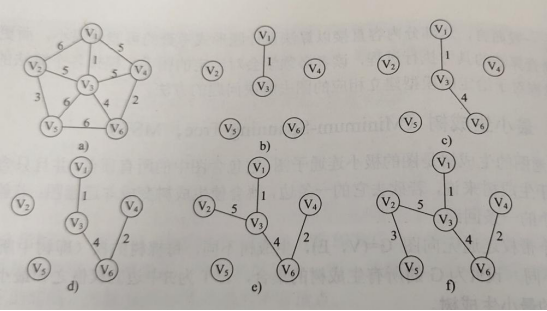简单伪代码实现如下：
void Prim(G,T)
{
T=NULL;   //初始化空树
U={w};    // 添加任意顶点
while((V-U)!=NULL)  //树中不含全部顶点即还不是生成树
{
T=T+(u,v);   //将权值最小的边加入树中
U=U+{v};   //顶点归入集合U中
}
}
克鲁斯卡尔(Kruskal)算法
Kruskal算法是一种按权值的递增次序选择合适的边来构造最小生成树的方法
算法思路：
（1）将图中的所有边都去掉。
（2）将边按权值从小到大的顺序添加到图中，保证添加的过程中不会形成环
（3）重复上一步直到连接所有顶点，此时就生成了最小生成树。这是一种贪心策略。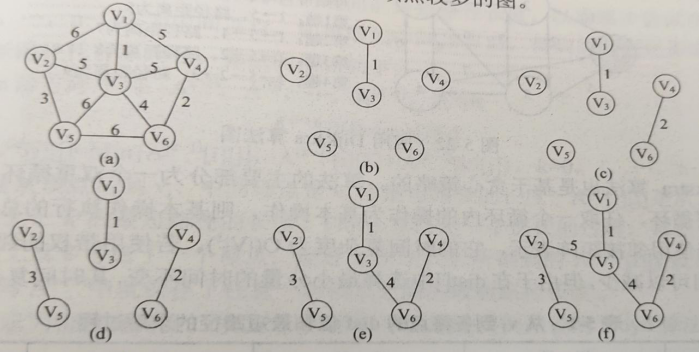Kruskal算法伪代码实现：
void Kruskal(V,T)
{
T=V;  //初始化树T，仅含顶点，也可以说仅含根结点的森林
numS=n;    //连通分量数
while(numS>1)
{
if((u,v)属于不同的连通分量)
{
T=T+(u,v);  //将权值最小的边加入生成树中
numS–;
}
}
}


展开全文• 最小生成树三个性质：最小生成树是树，因此其边等于顶点数减一，且树内一定不会有环对给定的图，其最小生成树可以不唯一，但边权之和一定唯一最小生成树是在无向图上面生成的，因此其根节点可以是这颗树上面的任意...
最小生成树最小生成树的定义：最小生成树是在一个给定的无向图G(V,E)中求一颗树T，使得这棵树拥有图G中所有顶点，且所有边都是来自图G中的边，并且满足整棵树的边权之和最小。最小生成树三个性质：最小生成树是树，因此其边数等于顶点数减一，且树内一定不会有环对给定的图，其最小生成树可以不唯一，但边权之和一定唯一最小生成树是在无向图上面生成的，因此其根节点可以是这颗树上面的任意一个节点。如果题目中涉及最小生成树的输出，为了让生成树唯一，一般会直接给出根节点，只需要给出节点作为根节点来求解最小生成树即可问题种类：铺设公路两种解决算法普利姆算法(prim)朴素版Prim算法(稠密图)，时间复杂度O(n^2)堆优化版Prim算法(稀疏图),时间 复杂度O(mlogn)(很少使用)克鲁斯卡尔算法(Kruskal)(稀疏图)朴素版prim算法假如有5个节点，6条边，A B 3A C 1B C 2A E 4C D 5C E 6T 表示当前包含所有节点的集合，U 表示NULL。假如从A开始，(将A加入到U中)第一步：选中A到各个节点中权重最小的节点第二步：判断该节点是否被访问过，如果没有被访问过，则将该节点加入到集合U中第三步：更新其他节点到集合U的距离第四步：选择到集合U最近的点，重复第二步时间复杂度是 $$O(n2+m)$$, n 表示点数，m 表示边数static int n;      // n表示点数static int INF = 0x3f3f3f3f;static int[][] g = new int[N][N];        // 邻接矩阵，存储所有边static int[] dist = new int[N];        // 存储其他点到当前最小生成树(集合)的距离static boolean st = new int[N];     // 存储每个点是否已经在生成树中// 如果图不连通，则返回INF(值是0x3f3f3f3f), 否则返回最小生成树的树边权重之和int prim(){Arrays.fill(dist, 0x3f);//所有生成树里的边的长度之和的最小值int res = 0;for (int i = 0; i < n; i ++ ){int t = -1;for (int j = 1; j <= n; j ++ ) {if (st[j] != false && (t == -1 || dist[t] > dist[j])) {t = j;}}if (i != 0 && dist[t] == INF) return INF;if (i != 0) res += dist[t];st[t] = true;for (int j = 1; j <= n; j ++ ) {dist[j] = min(dist[j], g[t][j]);}}return res;}对应题目/***prime算法实现* 输入样例：*      4 5*      1 2 1*      1 3 2*      1 4 3*      2 3 2*      3 4 4* 输出样例：*      6*/public class PrimAlgorithm {int INF = 500000;private Scanner sc = new Scanner(System.in);public void run() {int n = sc.nextInt();int m = sc.nextInt();int[][] graph = new int[n + 1][n + 1];for (int i = 0; i < n + 1; i++) {Arrays.fill(graph[i], INF);}for (int i = 0; i < m; i++) {int x = sc.nextInt();int y = sc.nextInt();int z = sc.nextInt();//无向图graph[x][y] = Math.min(graph[x][y], z);graph[y][x] = graph[x][y];graph[x][x] = 0;graph[y][y] = 0;}int res = prim(graph);if (res == INF) {System.out.println("impossible");} else {System.out.println(res);}}public int prim(int[][] graph) {// graph长度是n+1int n = graph.length - 1;// dist[i] 表示的是i到生成树集合的最小距离int[] dist = new int[n + 1];// 给dist初始化为无穷Arrays.fill(dist, INF);int res = 0;// 存储对应的边是否被访问过boolean[] st = new boolean[n + 1];// 因为之前存储graph的时候，是从下标1开始的，所以这里遍历时候就是从1开始for (int i = 1; i < n + 1; i++) {int t = -1;for (int j = 1; j < n + 1; j++) {if (st[j] == false && (t == -1 || dist[t] > dist[j])) t = j;}// 避免第一次循环的时候，直接返回if (i != 1 && dist[t] == INF) return INF;// 表示节点t已经被访问过st[t] = true;if (i != 1) {// 将节点添加到生成树中res += dist[t];//                System.out.println(res);}// 生成树是一个集合// 因为生成树添加了新的节点，所以更新其他未被访问的点到生成树的最小距离，for (int j = 1; j < n + 1; j++) {dist[j] = Math.min(dist[j], graph[t][j]);}}return res;}public static void main(String[] args) {new PrimAlgorithm().run();}}Kruskal算法前置知识：排序和并查集基本思想：按照权值从小到大的顺序选择n-1条边，并保证这n-1条边不构成回路。具体做法：首先构造一个只含n个顶点的森林，然后依权值从小到大从连通网中选择边加入到森林中，并使森林中不产生回路，直至森林变成一棵树为止。时间复杂度是 $$O(mlogm)$$, n 表示点数，m 表示边数思路：将所有边按权重w从小到大排序；枚举每条边的顶点a,bif (a,b 不在同一个集合中(并查集)) 将这条边加入集合中int n, m;       // n是点数，m是边数int[] p = new int[N];       // 并查集的父节点数组class Edge{     // 存储边int a, b, w;Edge(int a, int b, int c) {this.a = a;this.b = b; this.c = c;}};int find(int x){     // 并查集核心操作if (p[x] != x) p[x] = find(p[x]);return p[x];}public int kruskal(){Arrays.sort(edge, new Comparator() {@Overridepublic int compare(Edge o1, Edge o2) {if(o1.welse if(o1.w>o2.w)  return 1;else return 0;}});for (int i = 1; i <= n; i ++ ) p[i] = i;    // 初始化并查集int res = 0, cnt = 0;for (int i = 0; i < m; i ++ ) {int a = edges[i].a, b = edges[i].b, w = edges[i].w;a = find(a);b = find(b);if (a != b) {    // 如果两个连通块不连通，则将这两个连通块合并p[a] = b;res += w;cnt ++ ;}}if (cnt < n - 1) return INF;return res;}题目：/*** kruskal算法实现* 输入样例：*      4 5*      1 2 1*      1 3 2*      1 4 3*      2 3 2*      3 4 4* 输出样例：*      6*/public class KruskalAlgorithm {int INF = Integer.MAX_VALUE / 2;private Scanner sc = new Scanner(System.in);private int n;private int m;public static void main(String[] args) {new KruskalAlgorithm().run();}// 并查集private int find(int[] p, int x) {if (p[x] != x) p[x] = find(p, p[x]);return p[x];}private void run() {n = sc.nextInt();m = sc.nextInt();// 存储边List edgeList = new ArrayList<>();// 下标从1开始，因为节点的值是从1开始的int[] p = new int[n + 1];for (int i = 1; i <= n; i++) {p[i] = i;}for (int i = 0; i < m; i++) {int x = sc.nextInt();int y = sc.nextInt();int z = sc.nextInt();edgeList.add(new Edge(x, y, z));}int res = kruskal(edgeList, p);if (res == INF) {System.out.println("impossible");} else {System.out.println(res);}}private int kruskal(List edgeList, int[] p) {int res = 0;int cnt = 0;// 按照w 权重的大小来排序Collections.sort(edgeList);for (int i = 0; i < edgeList.size(); i++) {int a = edgeList.get(i).getA();int b = edgeList.get(i).getB();int w = edgeList.get(i).getW();// 分别找到a、b对应的集合的根a = find(p, a);b = find(p, b);// 如果根相同表示a、b在同一个集合中，如果添加进去，会形成回路，则该边不能添加到集合中// 如果根不相同表示a、b不在同一个集合中，则该边能添加到集合中if (a != b) {p[a] = b;res += w;// 计算添加进去的边cnt++;}}// n 是点的数量，n个点最少是n-1条边，如果少于n-1条边，则表示 n个点并没有全部使用到，所以不成立if (cnt < n - 1) return INF;return res;}}class Edge implements Comparable {int a, b, w;public Edge(int a, int b, int w) {// a,b 为节点this.a = a;this.b = b;// w 为节点之间的权重this.w = w;}public int getA() {return a;}public int getB() {return b;}public int getW() {return w;}// 设置edge的排序规则，按照w的大小排序@Overridepublic int compareTo(Edge edge) {return Integer.compare(w, edge.w);}}
展开全文• 最小生成树是树，因此其边数等于顶点数减1，且树内一定没有环； 对给定的图，其最小生成树不唯一，但边权之和是唯一的； 最小生成树是在无向图上生成的，因此其根结点可以是这个图上的任意个点。题目一般会指出...
详解: 最小生成树算法
最小生成树（Minimum Spanning Tree, MST）是在一个给定的无向图G(V, E)中求一棵树，使得这棵树有图G的所有顶点，且所有边都来自图G中的边，并且满足整棵树的边权之和最小.
最下生成树满足如下性质：

最小生成树是树，因此其边数等于顶点数减1，且树内一定没有环；
对给定的图，其最小生成树不唯一，但边权之和是唯一的；
最小生成树是在无向图上生成的，因此其根结点可以是这个图上的任意一个点。题目一般会指出那个点作为生成树的根结点

示例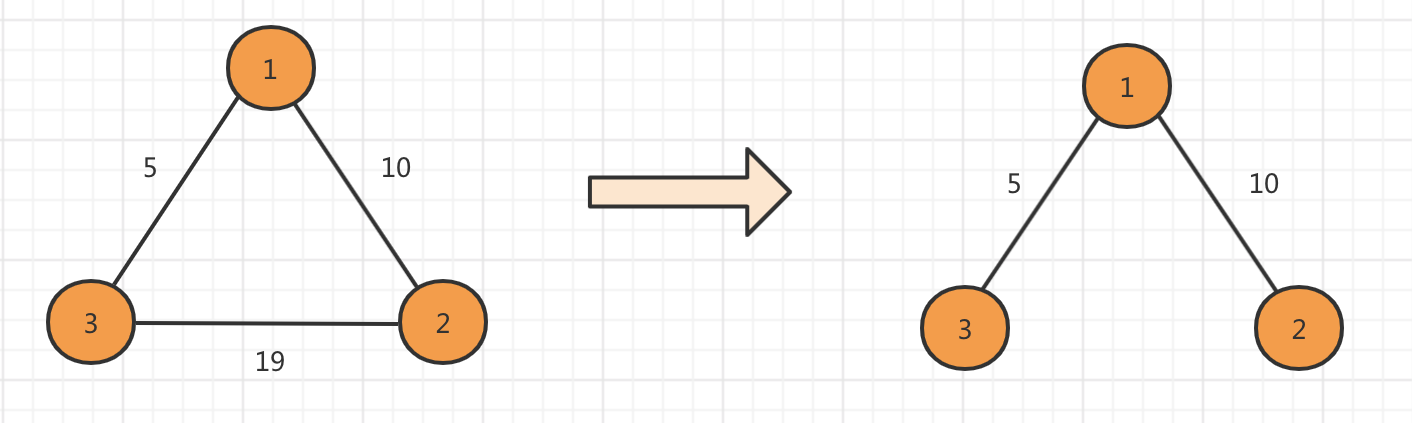输入
3 3
1 2 10
1 3 5
3 2 19
输出
15

Prim算法
跟Dijkstra算法类似，将顶点分为两个集合，一个是已经在生成树中的顶点集合S，另一个是还未访问的点。
用数组d[]表示各个顶点到集合S的最短距离（只有这里d的含义与Dijkstra算法中d的含义不一样）
Prim(G, d[]) {
初始化;
for (循环n次) {
u = 使d[u]最小的还未被访问的顶点的标号;
记u已被访问
for (从u出发能到达的所有顶点v){
if(v未被访问 && 以u为中介点使得v与集合S的最短距离d[v]更优){
将G[u][v]赋值给v与集合S的最短距离d[v]
}
}
}
}

基于邻接矩阵实现的Prim算法
Java
import java.util.Arrays;
import java.util.Scanner;

public class Main{

private static final int INF = Integer.MAX_VALUE;

public static void main(String[] args) throws InterruptedException {
Scanner input = new Scanner(System.in);
int n = input.nextInt();
int m = input.nextInt();
int[][] graph = new int[n + 1][n + 1];
for (int i = 0; i < n + 1; i++) Arrays.fill(graph[i], INF);
int u, v, w;
for (int i = 0; i < m; i++) {
u = input.nextInt();
v = input.nextInt();
w = input.nextInt();
graph[u][v] = graph[v][u] = w;
}
int start = 1; //指定一个结点作为生成树的根结点
int res = prim(graph, n, 1);
System.out.println(res);
}

public static int prim(int[][] graph, int n, int start) {
int[] d = new int[n + 1];
boolean[] visit = new boolean[n + 1];
Arrays.fill(d, INF);
d[start] = 0;
int ans = 0;
for (int i = 1; i <= n; i++) {
int u = -1, MIN = INF;
for (int j = 1; j <= n; j++) {
if (!visit[j] && d[j] < MIN) {
u = j;
MIN = d[j];
}
}
if (u == -1) return -1;
visit[u] = true;
ans += d[u]; // 将与集合S距离最小的边加入最小生成树
for (int v = 1; v <= n; v++) {
if (!visit[v] && graph[u][v] < INF && graph[u][v] < d[v]) {
d[v] = graph[u][v];
}
}
}
return ans;
}
}

C++
#include <iostream>
#include <vector>
using namespace std;

const int N = 100;
const int INF = INT_MAX;

int prim(vector<vector<int>>& graph, int n, int start) {
vector<int> d(n + 1, INF);
vector<bool> visit(n + 1, false);
d[start] = 0;
int ans = 0;
for (int i = 1; i <= n; i++) {
int u = -1, MIN = INF;
for (int j = 1; j <= n; j++) {
if (!visit[j] && d[j] < MIN) {
u = j;
MIN = d[j];
}
}
if (u == -1) return -1;
visit[u] = true;
ans += d[u];
for (int v = 1; v <= n; v++) {
if (!visit[v] && graph[u][v] < INF && graph[u][v] < d[v]) {
d[v] = graph[u][v];
}
}
}
return ans;
}

int main() {
int n, m;
cin >> n >> m;
vector<vector<int>> graph(n + 1, vector<int>(n + 1, INF));
int u, v, w;
for (int i = 0; i < m; i++) {
cin >> u >> v >> w;
graph[u][v] = graph[v][u] = w;
}
int start = 1;
int res = prim(graph, n, start);
cout << res << endl;
return 0;
}

基于邻接表实现的Prim算法
Java
import java.util.ArrayList;
import java.util.Arrays;
import java.util.List;
import java.util.Scanner;

public class Main{

private static final int INF = Integer.MAX_VALUE;

public static void main(String[] args) throws InterruptedException {
Scanner input = new Scanner(System.in);
int n = input.nextInt();
int m = input.nextInt();
List<List<Node>> graph = new ArrayList<>(n + 1);
for (int i = 0; i < n + 1; i++) graph.add(new ArrayList<>());
int u, v, w;
for (int i = 0; i < m; i++) {
u = input.nextInt();
v = input.nextInt();
w = input.nextInt();
graph.get(u).add(new Node(v, w));
graph.get(v).add(new Node(u, w));
}
int start = 1;
int res = prim(graph, n, start);
System.out.println(res);
}

public static int prim(List<List<Node>> graph, int n, int start) {
int[] d = new int[n + 1];
boolean[] visit = new boolean[n + 1];
Arrays.fill(d, INF);
d[start] = 0;
int ans = 0;
for (int i = 1; i <= n; i++) {
int u = -1, MIN = INF;
for (int j = 1; j <= n; j++) {
if (!visit[j] && d[j] < MIN) {
u = j;
MIN = d[j];
}
}
if (u == -1) return -1;
visit[u] = true;
ans += d[u];
for (int k = 0; k < graph.get(u).size(); k++) {
int v = graph.get(u).get(k).v; //从u能到达的顶点v
if (!visit[v] && graph.get(u).get(k).dis < d[v]) {
d[v] = graph.get(u).get(k).dis;
}
}
}
return ans;
}

static class Node{
int v, dis;
public Node(int v, int dis) {
this.v = v;
this.dis = dis;
}
}
}

C++
#include <iostream>
#include <vector>
using namespace std;

const int N = 100;
const int INF = INT_MAX;

struct Node{
int v, dis; //v为边的目标顶点，dis为边权
Node(int _v, int _dis): v(_v), dis(_dis) {}
};

int prim(vector<vector<Node*>>& graph, int n, int start) {
vector<int> d(n + 1, INF);
vector<bool> visit(n + 1, false);
d[start] = 0;
int ans = 0;
for (int i = 1; i <= n; i++) {
int u = -1, MIN = INF;
for (int j = 1; j <= n; j++) {
if (!visit[j] && d[j] < MIN) {
u = j;
MIN = d[j];
}
}
if (u == -1) return -1;
visit[u] = true;  // 将u放入集合S中
ans += d[u];
// 遍历从u能到达的顶点，更新它们到达集合S的最短距离
for (int k = 0; k < graph[u].size(); k++) {
int v = graph[u][k]->v; //从u能到达的顶点
if (!visit[v] && graph[u][k]->dis < d[v]) {
d[v] = graph[u][k]->dis;
}
}
}
return ans;
}

int main() {
int n, m;
cin >> n >> m;
vector<vector<Node*>> graph(n + 1);
int u, v, w;
for (int i = 0; i < m; i++) {
cin >> u >> v >> w;
graph[u].push_back(new Node(v, w));
graph[v].push_back(new Node(u, w));
}
int start = 1;
int res = prim(graph, n, start);
cout << res << endl;
return 0;
}

Kruskal算法
采用边贪心的策略，思想很简单：

对所有边权按从小到大进行排序；
按边权从小到大测试所有边，如果当前测试边所连接的两个顶点不在同一个连通块中，则把这条边加入当前最小生成树中；否则，将边舍弃；
执行步骤2，直到最小生成树中的边数等于总顶点数减1或是测试完所有边时结束。而当结束时如果最小生成树中的边数小于总顶点树减1，说明该图不连通.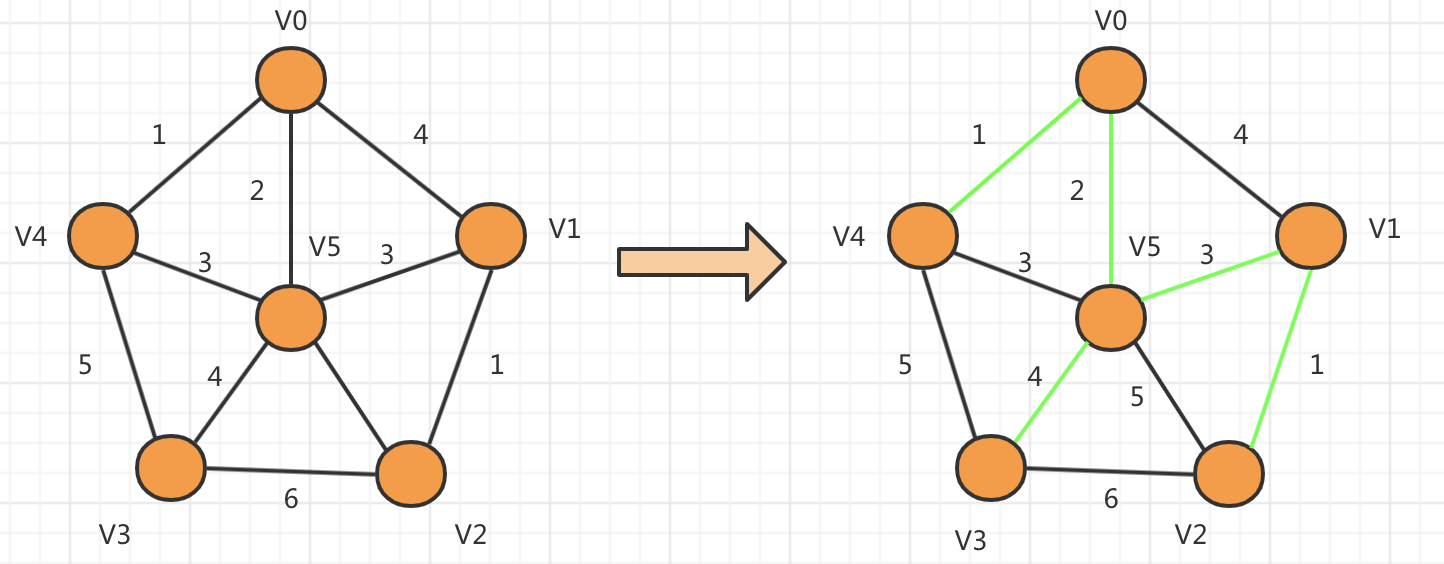int kruskal() {
令最小生成树的边权之和为 ans, 最小生成树的当前边数为Num_Edge;
将所有边按边权从小到大排序;
for (从小到大枚举所有边){
if (当前测试边的两个端点在不同的连通块中) {
将测试边加入最小生成树中;
ans += 测试边的边权;
最小生成树的当前边数Num_Edge加1;
当边数等于顶点数减1时结束循环;
}
}
return ans;
}

伪代码中有两个细节私护不太直观，即：

如何判断测试边的两个端点在不同的连通块中；
如何将测试边加入最小生成树中。

把每个连通块当作一个集合，那么就可以把问题转换为判断两个端点是否在同一个集合中，这正好可以使用并查集。
并查集可以通过查询两个结点所在集合的根结点判断是否来自同一集合；
将测试边加入连通块可以通过将两个端点所在集合合并，也正好利用了并查集的合并特性；
Java
import java.util.Arrays;
import java.util.Comparator;
import java.util.Scanner;

public class Main{
public static int[] father;
public static void main(String[] args) {
Scanner input = new Scanner(System.in);
int n = input.nextInt();
int m = input.nextInt();
Edge[] edges = new Edge[m];
father = new int[n + 1];
for (int i = 0; i < n; i++) father[i] = i;
int u, v, w;
for (int i = 0; i < m; i++) {
u = input.nextInt();
v = input.nextInt();
w = input.nextInt();
edges[i] = new Edge(u, v, w);
}
int res = kruskal(edges, n, m);
System.out.println(res);
}

public static int kruskal(Edge[] edges, int n, int m) {
Arrays.sort(edges, new Comparator<Edge>() {
@Override
public int compare(Edge o1, Edge o2) {
if (o1.w > o2.w) return 1;
else if (o1.w == o2.w) return 0;
else return -1;
}
});
int ans = 0;
int numEdges = 0;
for (int i = 0; i < m; i++) {
int faU = findFather(edges[i].u);
int faV = findFather(edges[i].v);
if (faU != faV) {
ans += edges[i].w;
father[faU] = faV;
numEdges++;
if (numEdges == n - 1) break;
}
}
if (numEdges != n - 1) return -1;
else return ans;
}

public static int findFather(int a) {
int x = a;
while (x != father[x]) {
x = father[x];
}
// 压缩路径
while (a != father[a]) {
int z = a;
a = father[a];
father[z] = x;
}
return x;
}

static class Edge{
int u, v, w;
public Edge(int u, int v, int w) {
this.u = u;
this.v = v;
this.w = w;
}
}
}

C++
#include <iostream>
#include <vector>
using namespace std;

const int MAXV = 100;
const int MAXE = 100;

struct edge{
int u, v; //边的两个断点
int cost; //边权
}E[MAXE];  //最多有MAXE条边

bool cmp(edge a, edge b) {
return a.cost < b.cost;
}

// 并查集部分
int father[MAXV]; //并查集数组
int findFather(int x) { //并查集查询函数
int a = x;
while (x != father[x]) {
x = father[x];
}
// 路径压缩
while (a != father[a]) {
int z = a;
a = father[a];
father[z] = x;
}
return x;
}

// 返回最小生成树的边权之和，参数n为顶点个数，m为图的边数
int kruskal(int n, int m) {
int ans = 0, Num_Edge = 0;
for (int i = 0; i < n; i++) {
father[i] = i;
}
sort(E, E + m, cmp);
for (int i = 0; i < m; i++) { //枚举所有边
int faU = findFather(E[i].u);
int faV = findFather(E[i].v);
if (faU != faV) { // 不在同一个连通块，将边加入最小生成树
father[faU] = faV;
ans += E[i].cost;
Num_Edge++;
if (Num_Edge == n - 1) break;
}
}
if (Num_Edge != n - 1) return -1; //无法连通返回-1
else return ans;
}

int main() {
int n, m;
cin >> n >> m;
int u, v, w;
for (int i = 0; i < m; i++) {
cin >> u >> v >> w;
E[i].u = u;
E[i].v = v;
E[i].cost = w;
}
int res = kruskal(n, m);
cout << res << endl;
return 0;
}



展开全文算法 数据结构
• 最小生成树的定义： 在一给定的无向图G=（U，V）中,（U，V）代表链接顶点U，V的边，而W（U，V）代表此边...最小生成树的边数必然是顶点数减一，|E| = |V| - 1。 最小生成树不可以有循环。 最小生成树不必是唯一
• 文章目录1 概念1.1 定义1.2 性质2 求解最小生成树2.1 prim算法（普里姆算法） ...1 最小生成树是树，因此其边等于其顶点减一，且树内一定不会有环； 2 对于给定的图G(V,E),其最小生成树可以不唯...
• 1、最小生成树是树，其边数等于顶点数减1，且不会有环 2、对于给定的图最小生成树可以不唯一，但是边权之和一定是唯一的。 3、其根节点可以是这棵树上的任何个节点，题目中不做说明这默认根节点是0号顶点是根...
• 最小生成树 定义 生成树：连通图包含全部顶点的个极小连通子图 ...最小生成树的边数为顶点数减1 算法 Prim 算法适用于稠密图，Kruskal算法适用于稀疏图 Prim 算法 初始化：向空的结果树 T=(VT,ET数据结构与算法
• ## 最小生成树算法

千次阅读 2013-11-20 14:27:18
1.最小生成树的概念 在给定的无向图G = (V, E) 中，(u, v) 代表连接顶点 u 与顶点 v 的边（即），而 w(u, v) 代表此边的权重，若存在 T 为 E 的子集（即）且为无循环图，使得 ...最小生成树的边数必然是顶点数减算法
• ## 图论 最小生成树

千次阅读 2011-11-28 21:35:45
最小生成树：在一给定的无向图 G = (V, E) 中，(u, v) 代表连接顶点 u 与顶点 v 的边（即 ），而 w(u, v) 代表此边的权重，若存在 T 为 E 的子集（即 ...最小生成树的边数必然是顶点数减一，|E| = |V| - 1。最小生成树算法 数据结构 c
• 图的应用主要包括最小生成树，最短路径，拓扑排序和关键路径，这些知识点是考研的重要考点...最小生成树不唯一，但是权值和是唯一确定的，另外最小生成树的边数是顶点数减一最小生成树算法有prim算法，prim算法 类...
• 先上最小生成树定义： 在一个给定的无向图G=(V,E)中， (u,v)代表连接顶点u和v...最小生成树的边数必然是顶点数减一，|E| = |V| - 1。 最小生成树不可以有循环。 最小生成树不必是唯一的。 求解一个图的最小生...
• 最小生成树是树，因此其边数等于顶点数减1，且树内一定不会有环 ②对给定的图G(V,E),其最小生成树可以不唯一，但其边权之和一定是唯一的 ③由于最小生成树是在无向图上生成的，因此其根节点可以是这棵树上的任意...贪心算法 队列 算法
• 文章目录概念定义性质算法Prim想法算法代码...最小生成的边数是顶点数减1 算法 基本想法：贪心法——每一步做出最好的选择 基本思想： GENRIC_MST(G){ T=NULL; while T 未形成生成树： do 找到条最小代数据结构
• 最小生成树是树，因此其边数是等于顶点数减1，而且树内一定不会有环 ② 对于给定的图G（V，E）其最小生成树可以是不唯一的，但是边权这个是唯一的 ③ 由于最小生成树是在无向图中产生的，所...
• 最小生成树 文章目录最小生成树Prim算法基本思想具体实现邻接矩阵版邻接表版Kruskal 算法基本思想具体实现 最小生成树（Minimum Spanning Tree，MST）是在个给定的无...最小生成树是树，因此其边数等于顶点数减 1...数据结构 算法 c++
• 最小生成树定义： 一个无向图，任意两个顶点...注意：一个完整的最小生成树只需要顶点数减一条边 先来讲克鲁斯卡尔： 把边的权值，从小到大查看一遍，如果不产生圈，就把当前边加入生成树中。 如何判断是否产...
• 最小生成树是树，因此其边等于顶点数减一，且树内一定不会有环 ②对给定的图，其最小生成树可以不唯一，但其边权之和一定是唯一的 ③由于最小生成树是在无向图上生成的，因此其根结点可以是这棵书上的任意一个...
• 个连通图的生成树是图的极小连通子图。它包含图中的所有顶点，并且只含尽可能少的边。...3.最小生成树的边数为顶点数减1。 构造最小生成树可以有多种算法。其中多数算法利用了最小生成树的下列邻接矩阵
• 1.最小生成树边等于顶点数减一，且内不会有环； 2.对给定的G(V,E)，其最小生成树可以不唯一，但其边权之和是唯一的； 3.最小生成树无向图上生成的，因此其根节点可以这棵上的任意节点。 2.prim算法思想： ...
• 最小生成树是树，因此其边数等于顶点数减1，且树内一定不会有环。 对于给定的图G，其最小生成树可以不唯一，但其边权之和一定是唯一的 由于最小生成树是在无向图上生成的，因此其根结点可以实这棵树上的任意个结点...
• 对于个连通图而言，若存在子图包含原图全部顶点且边的条数为顶点数减1 那么这个子图就叫做原图的生成树 什么叫做最小生成树呢 ，就是说在生成树的前提下，其边上的权值之和最小的生成树就叫做最小生成树 如图所...java mst
• 最小生成树的克鲁斯卡尔(Kruskal)算法实现主要是通过将输入的图的边存在优先队列中，然后依次从优先队列中取最小的边加入生成树，最小生成树的边的个数是顶点数减一，因此退出条件是while (!pq.empty() || cnt < ...数据结构 算法 c++
• 由于数据结构学过段时间了，有些知识点有点遗忘，所以先来复习一下...1，最小生成树是树，因此其边数等于顶点数减1，且树内一定不会有环。 2，对于给定的图G，其最小生成树可以不唯一，但其边权之和一定是唯一的 ...算法
• 转载自C++ 最小生成树之Prim（普里姆）算法 ...最小生成树是树.边是点数1.树中不存在环. 最小生成树不唯一.但是权值一定相同. 根节点可以是任意个节点. 基本思想 对图G（V,E）设置集合S，存放已经被访问...